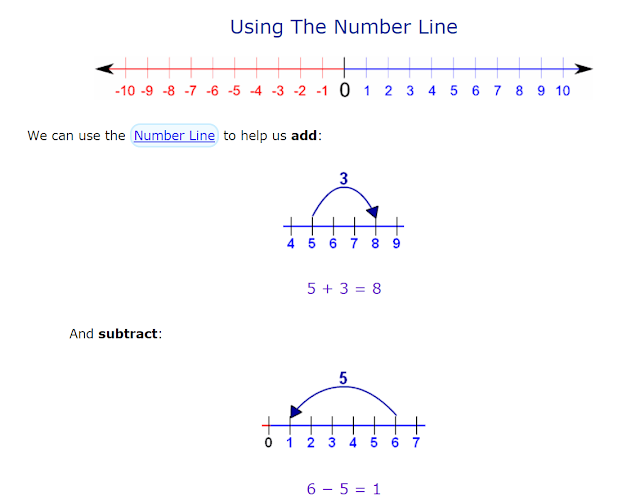### What Is Necessary for a Child to Learn Arithmetic

Adding, subtracting, multiplying and dividing whole numbers are the essentials of arithmetic. The next level in elementary schools involves fractions and decimals. When students are struggling in elementary mathematics it maybe useful to investigate what abilities correlate with the skills that are important in elementary mathematics. A recent study focusing on at risk fourth grade students from a southeastern metropolitan school district in the United States offers some clues.

The research work, Cognitive Predictors of Calculations and Number Line Estimation With Whole Numbers and Fractions Among At-Risk Students, first looks at the following measures.

• Incoming calculation skills
• Nonverbal reasoning
• Language
• Concept formation
• Working memory (numerical)
• Working memory (sentences)
• Processing speed
• Attentive behavior
The authors then examine which one(s) correlate with a student's performance in whole number calculation and representation on the number line.Above copied from Math is Fun
Likewise, the same investigation is performed on fractions, calculations and their number line representation.Above copied from Learn Zillion

And the results of the study are as follows:

Cognitive factors that correlate with whole number calculations:
• Incoming calculation skills
• Processing speed
• Attentive behavior
Adding and subtracting double-digit numbers clearly depend on fluency and attention.

Cognitive factors that correlate with representing a whole number on a number line:
• Nonverbal reasoning
• Working memory (numerical)
The lack of overlap between number line representation and calculation indicates that these two exercises maybe requiring different abilities from a child.

Cognitive factors that correlate with calculations involving fractions:

• Processing speed
• Attentive behavior
• Language
Here, incoming skills in whole number calculations do not correlate with fractions. Instead, language, primarily vocabulary and oral comprehension, now seems to matter.

Cognitive factors that correlate with fraction representation on a number line:
• Language
• Nonverbal reasoning
Similar to whole numbers, nonverbal reasoning, which includes nonverbal fluid reasoning, spatial ability, and perceptual organization with pattern completion, classification, analogy, and serial reasoning, appears to be consequential.

These findings can help teachers design interventions especially in the case of children who are struggling in math. For instance, learning how to calculate clearly requires attentive behavior. Teachers should therefore try to promote students' on task behavior and engagement in these lessons. Language seems crucial with fractions so it maybe helpful to use simple and clear words when discussing fractions. Mathematical abilities with both whole numbers and fractions obviously correlate with performance in math subjects in high school. It is thus important to address these challenges early on.